CIM Created Edited

# [CIM/Infor-Base] After Assigning Girder to Rigid Path, Assembly Unit is Not Assigned to Layout.

## Question

After assigning the girder to the Rigid Path, the Assembly Unit is not assigned to the Layout.

Reset the Coincident Point of the girder arrangement.

Info

First, the types of constraint paths used in CIM are
Main Constraint Path, Rigid Constraint Path, and Sub Constraint Path. All Constraint Paths regenerate paths when assigned to a parent mode.
Among them, the  Rigid Constraint Path  is a straight path that is dependent on the existing Constraint Path and maintains a specific length that is regenerated.
Therefore, Rigid Constraint Path is used for structures that change according to the alignment like PSC Beam of the bridge, but need to maintain a specific length.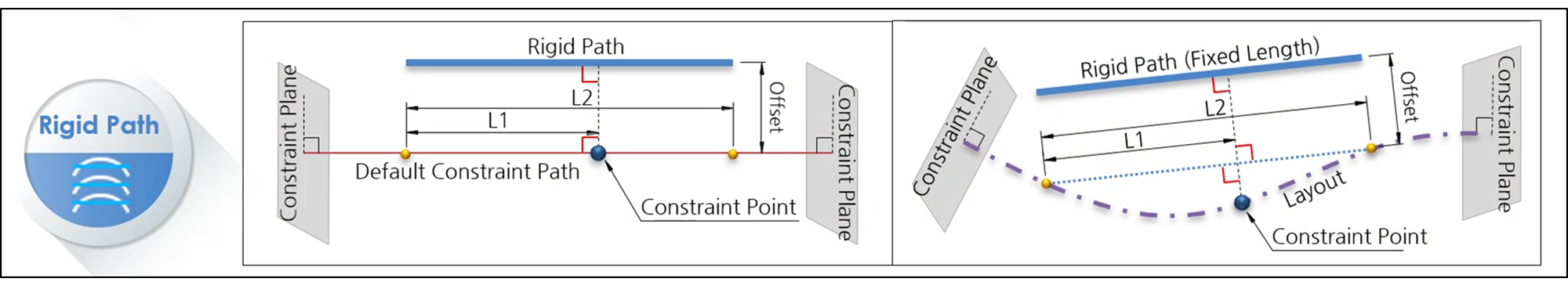Figure. 1 Rigid Constraint Path Concept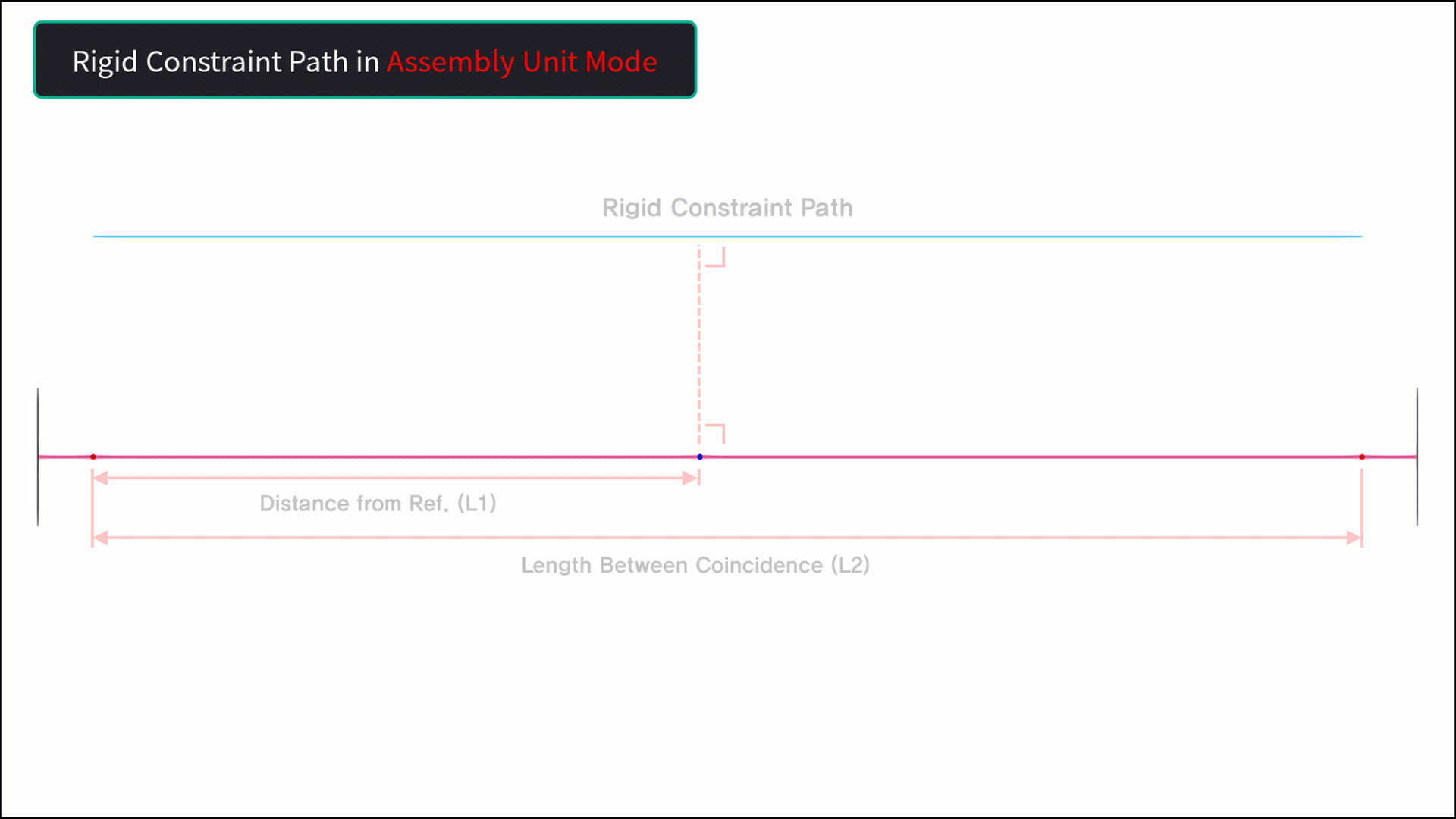Figure. 2 Assign upper mode of Rigid Constraint Path
Info

Let's look at the properties of Rigid Constraint Path a little more.
The properties of Rigid Constraint Path are as shown in <Figure.3> below, and among them, the property that affects the regeneration of Rigid Constraint Path is Coincident Point.
Therefore, the Rigid Constraint Path can be regenerated in the upper mode only when the Length information for this Coincident Point is entered correctly.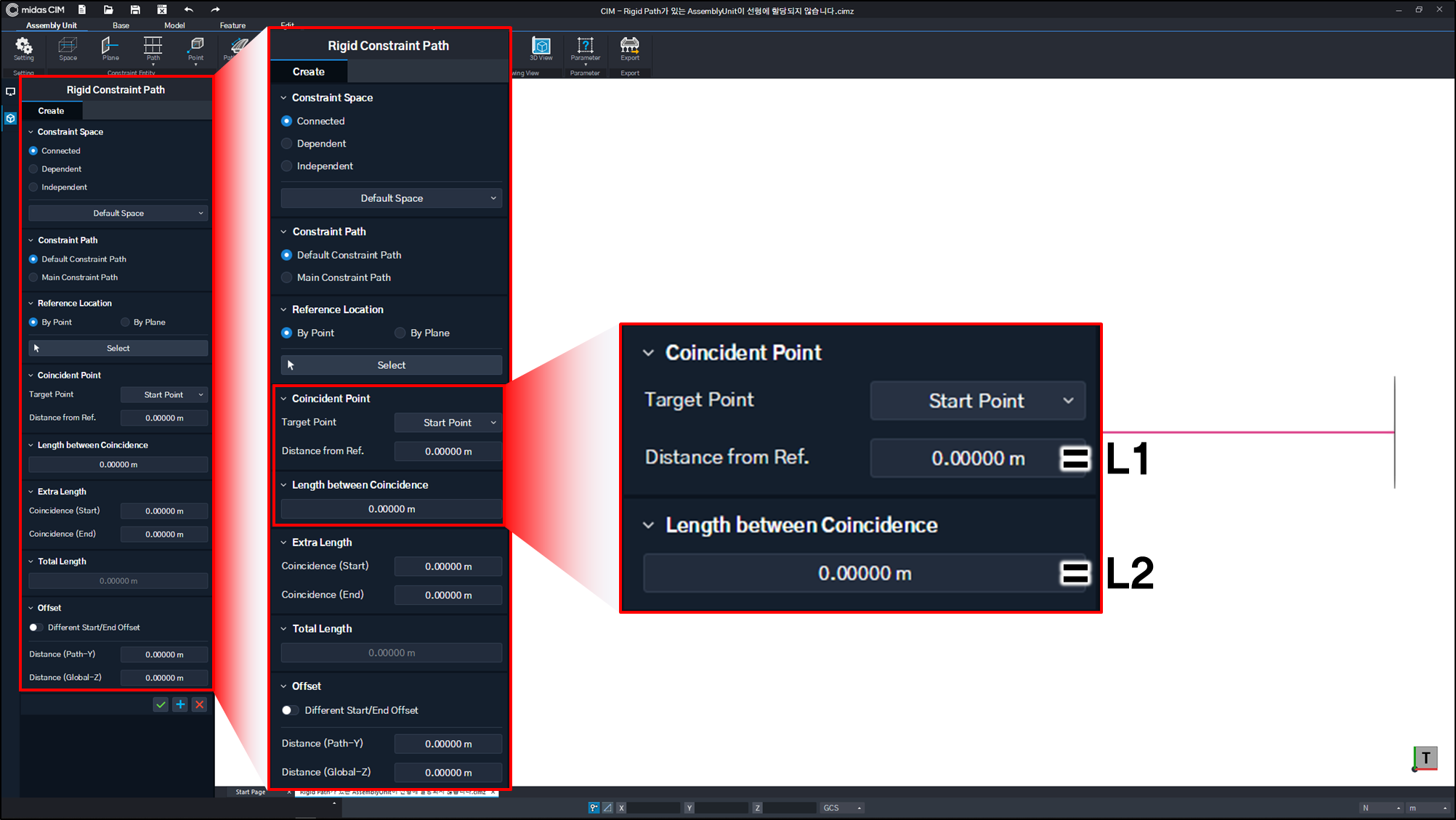Figure. 3 Coincident Point Property

Here, after the Distance from Ref. and Length between Coincidence of Coincident Point are L1 and L2, respectively,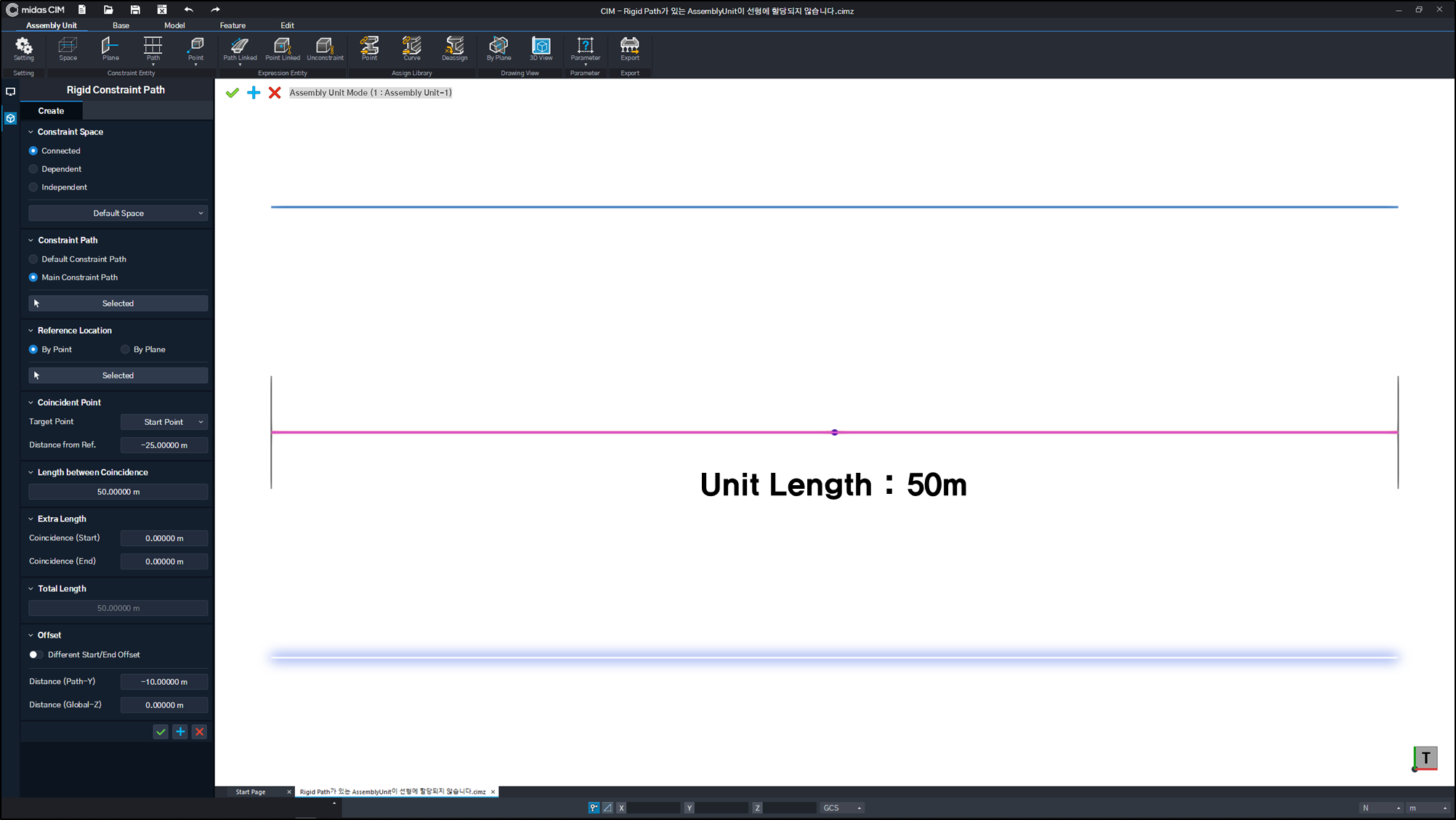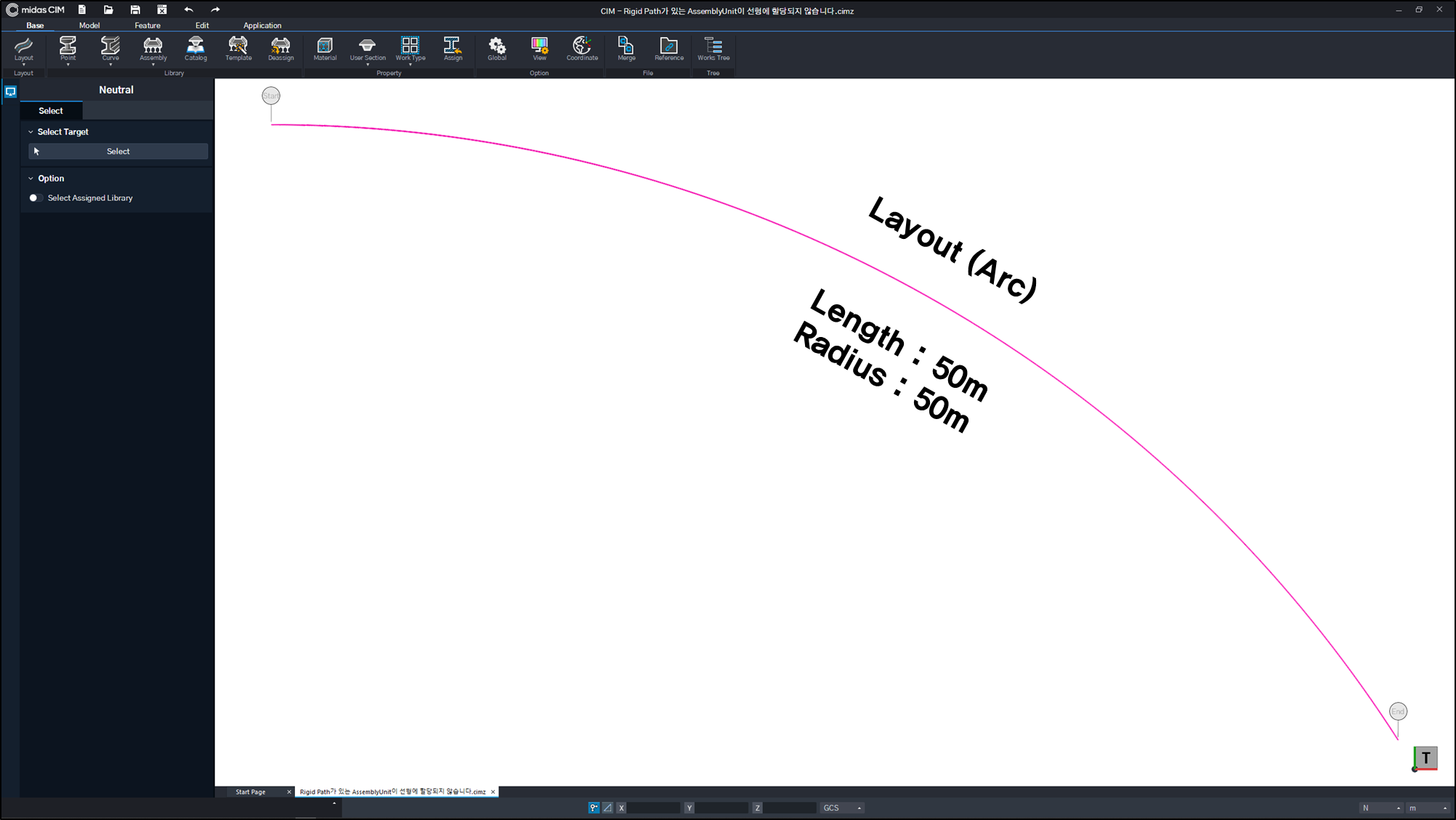Figure. 4 Assembly Unit Mode Figure. 5 Base Mode

1. Example of Rigid Constraint Path creation failure

Picture below. 6, to make a 50m rigid constraint path, define L1 and L2 as 25m and 50m, respectively.

However, the maximum straight line distance that can be found in a curved line with a length of 50 m is <Fig. As in 7>, it is about 47m The straight line distance between Coincident Points defined by L1 and L2 is 50m.

Therefore, since it is not possible to find a Coincident Point with a straight line distance of 50 m in a curved line, Rigid Constraint Path cannot be assigned to parent mode.
(Distance between Coincident Points defined by L1 and L2 > Maximum straight line distance in alignment)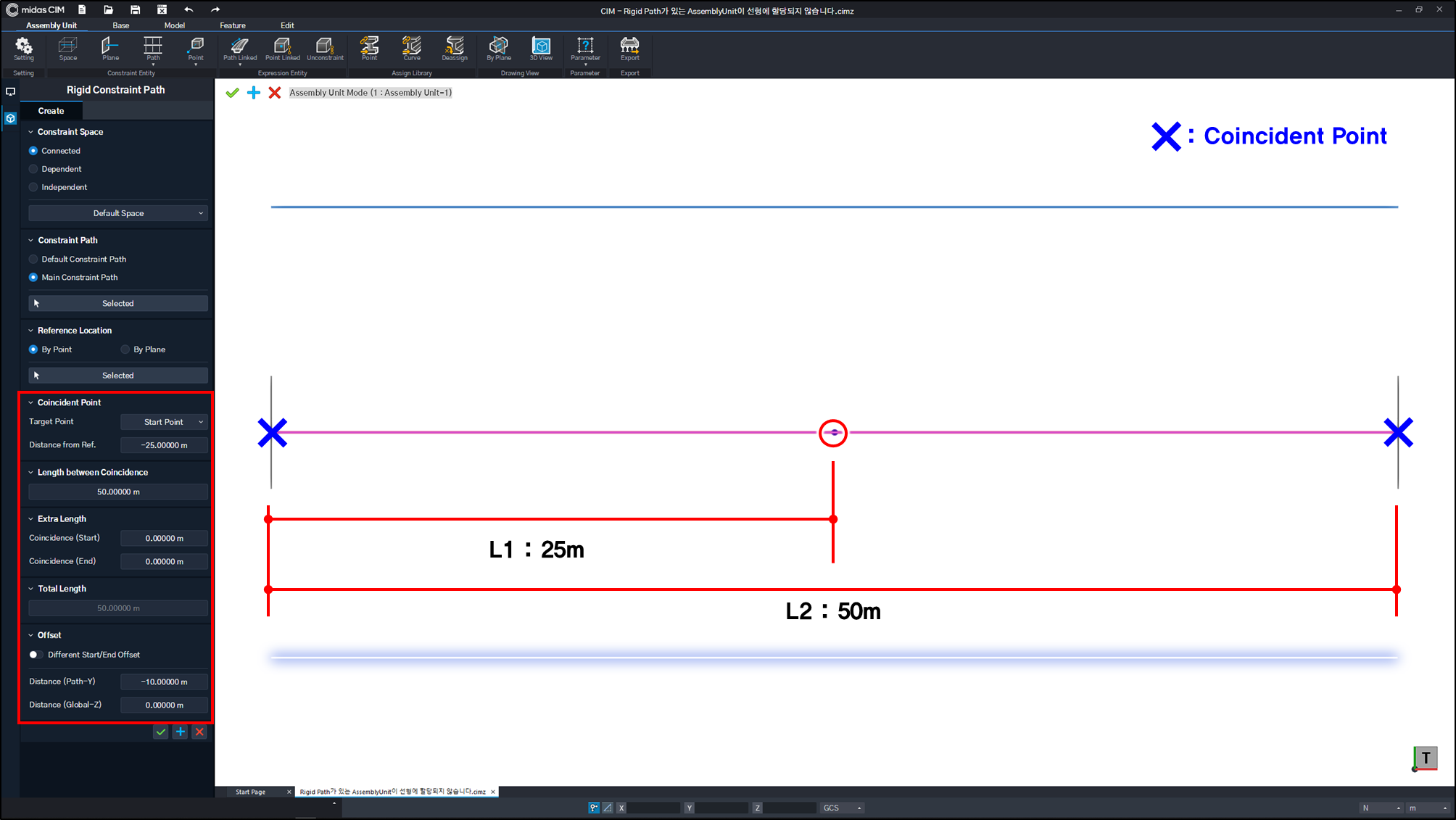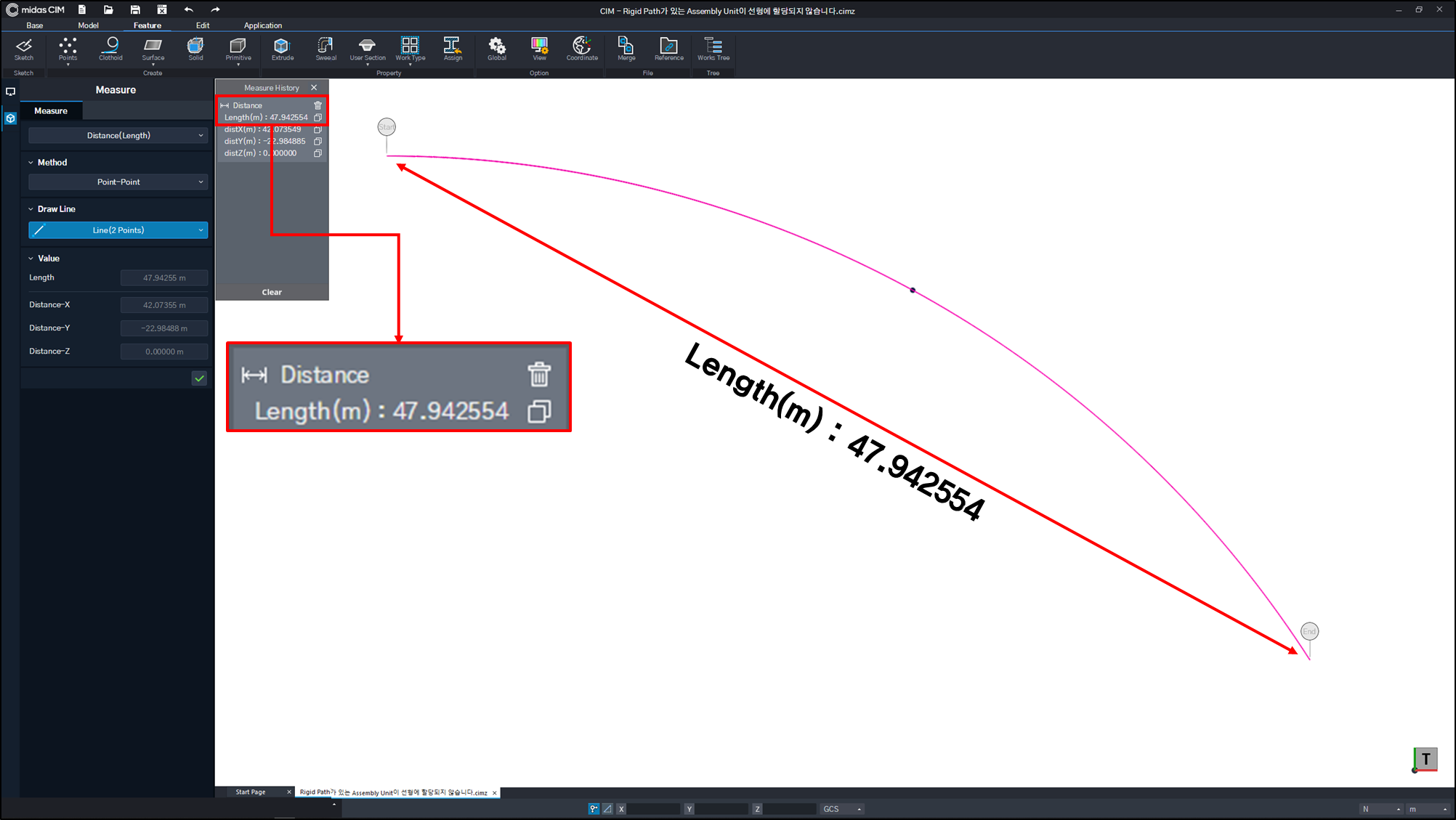Figure 6. When L1=25m, L2=50m Figure. 7 Rigid Constraint Path creation failed(L1, L2 distance insufficient)

2. A good example of creating a Rigid Constraint Path

Figure. Let's assume that it was created using Extra Length as shown in 8. Assuming that the bridge bearing is located 2m from the end of the girder The position of the bridge bearing is Coincident Point, This is an example of setting the distance from the bridge bearing to the end of the girder as Extra Length. (Refer to Figure. 9)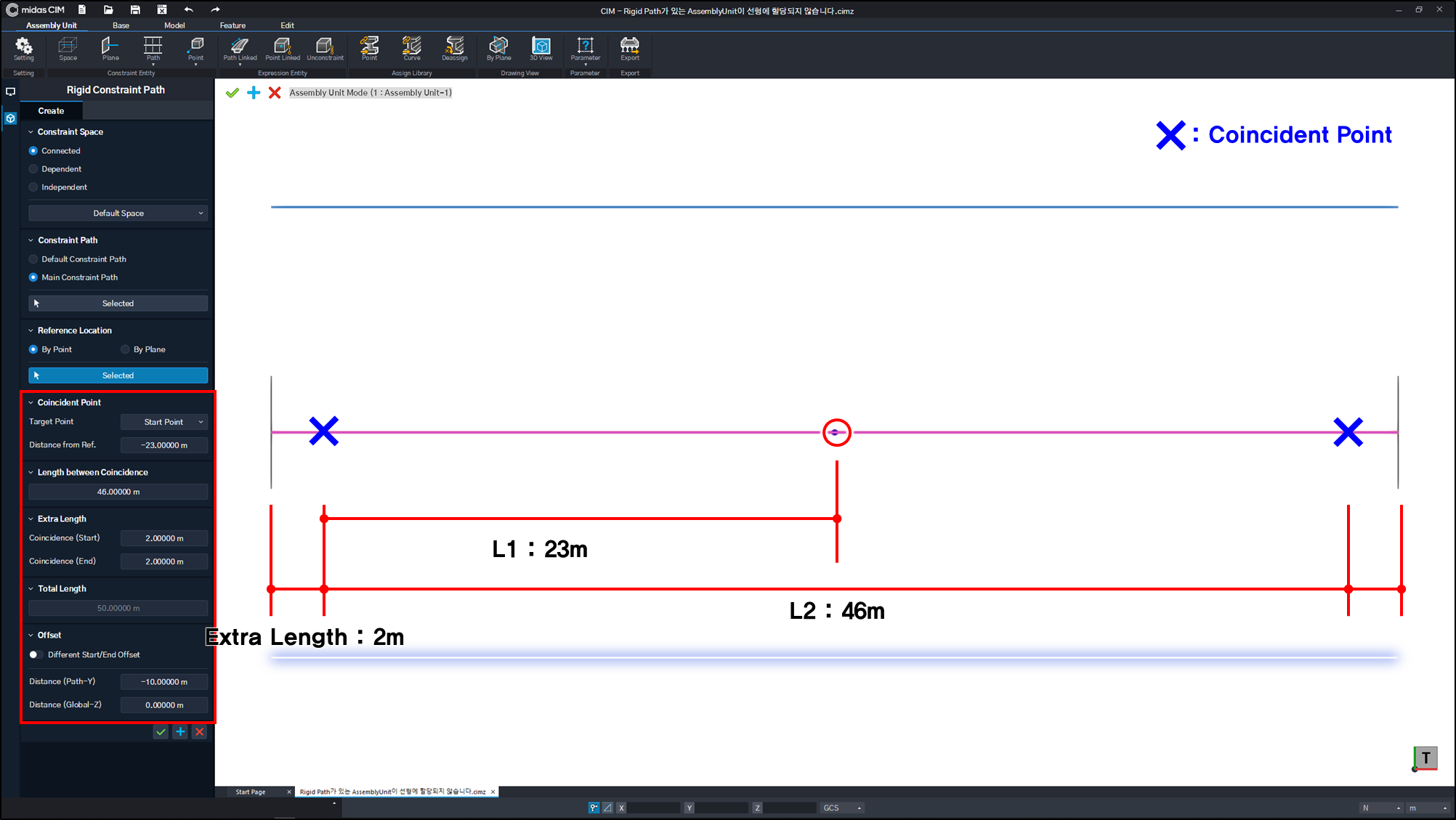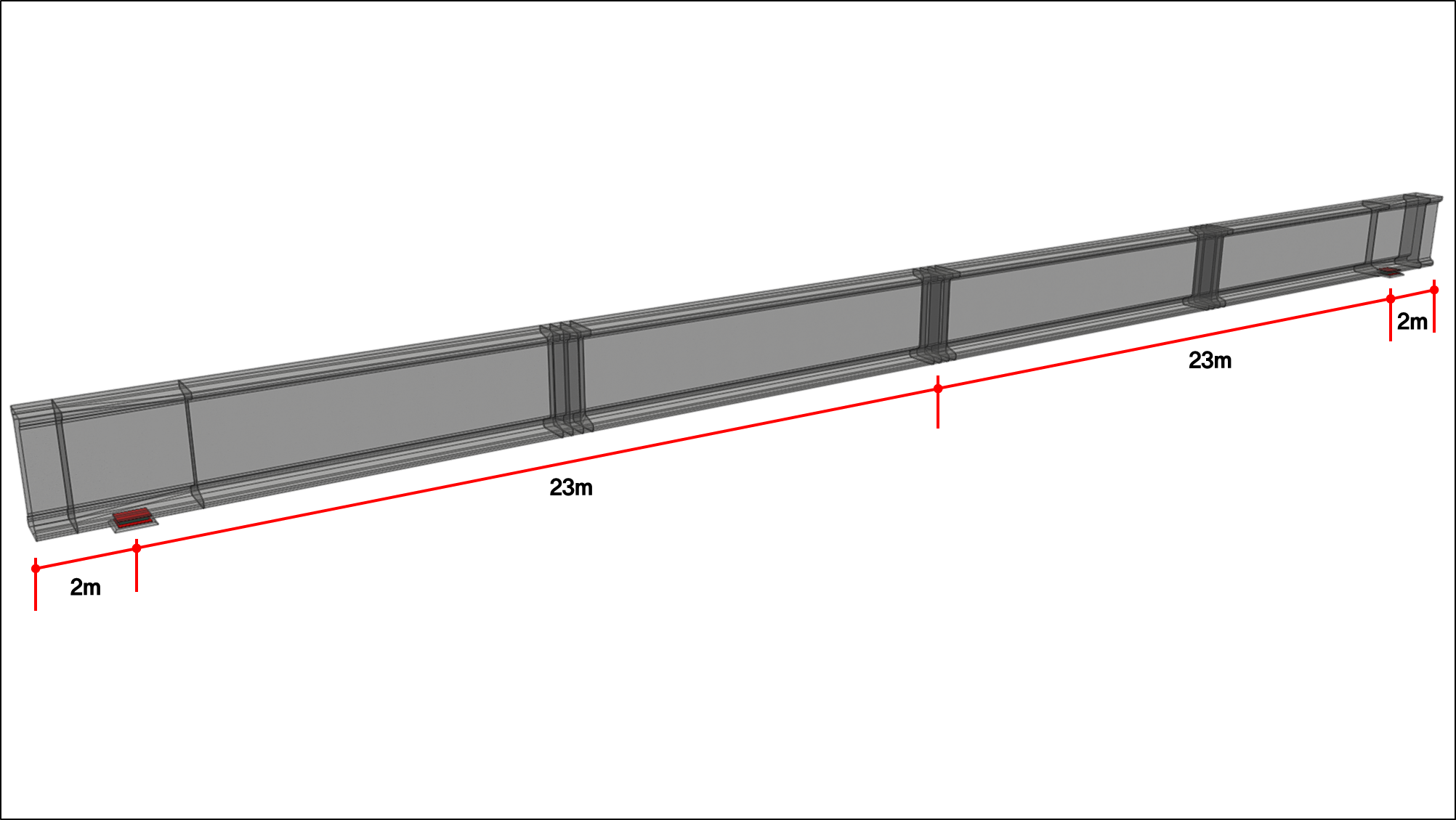figure. 8 Create Rigid Constraint Path figure. 9 When the Coincident Point position is set to the bridge support position

The Rigid Constraint Path created in this way is (Figure. 10) as Since the distance between Coincident Points is 46m, Because it is shorter than the maximum straight line distance of 47 m that can be found in a curved line of 50 m, Coincident Point can be found in Layout in upper mode.
(Distance between Coincident Points defined by L1 and L2 ≤ Maximum alignment distance in alignmnet)

Therefore, the Rigid Constraint Path can be assigned to the upper mode and An Assembly Unit with a girder assigned to the Rigid Constraint Path can also be created in the upper mode. (Refer to Figure. 11)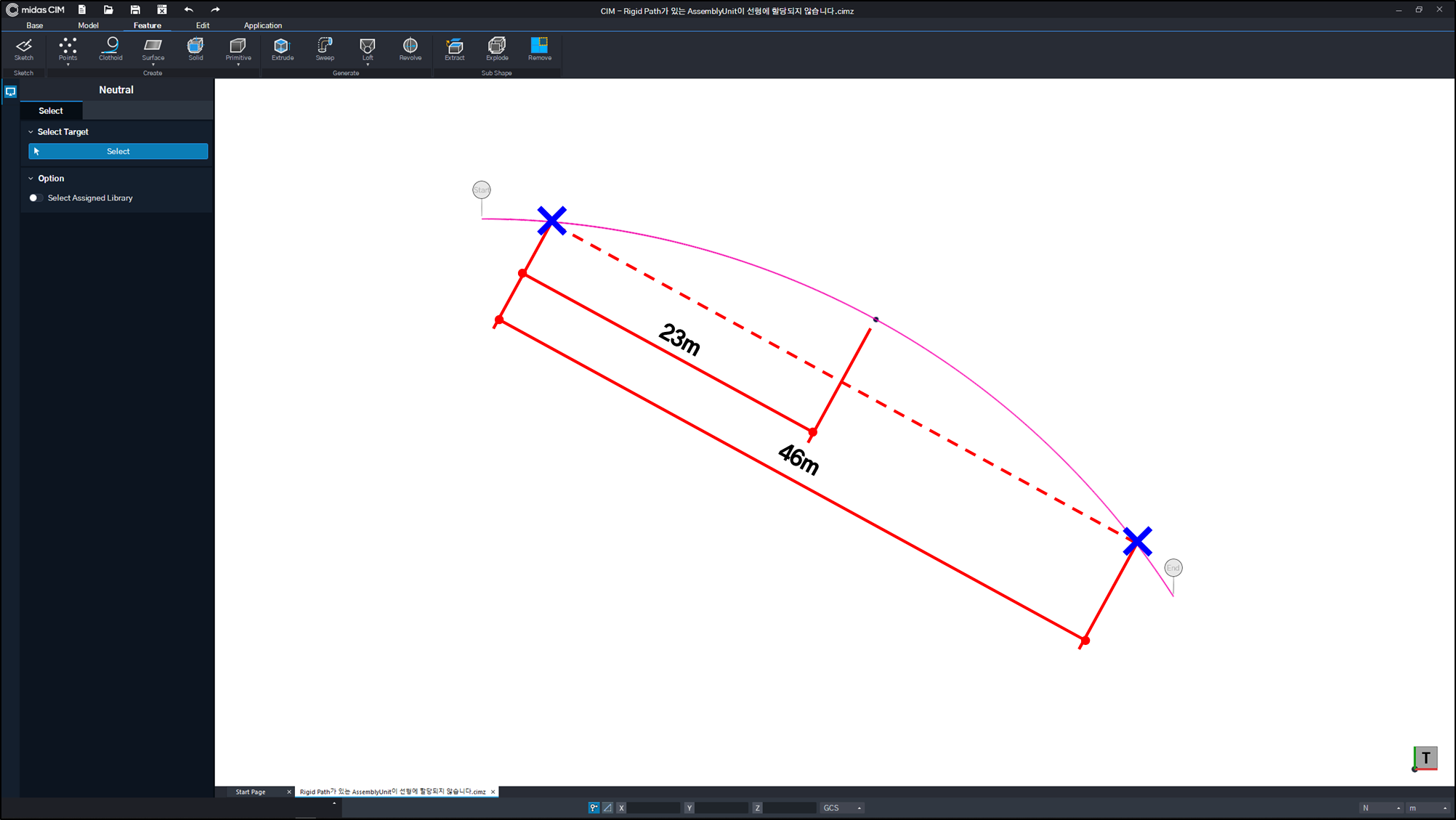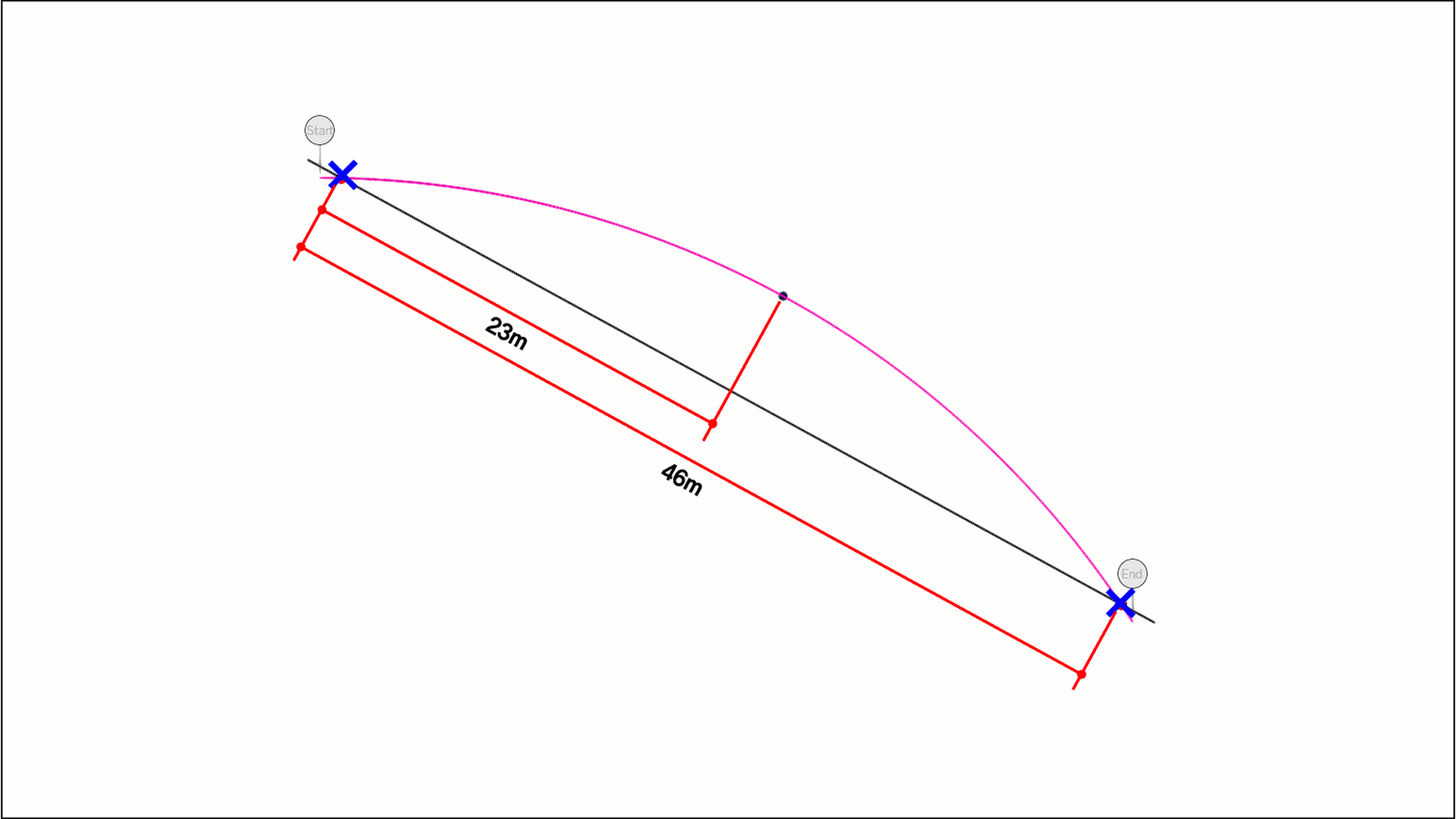Figure. 10 Layout assignment of Rigid Constraint Path Figure. 11 Process of assigning rigid constraint path to alignment

To sum up,

Just like setting the property by setting the base of girder arrangement (Coincident Point) to the position of the bridge bearing rather than the end point of the girder.
We recommend that you decide Coincident Point according to the situation according to the judgment of the designer.

0